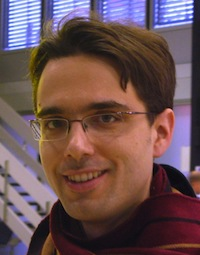Vedran Sohinger - University of Pennsylvania Mathematics

# Vedran Sohinger

#### Simons Postdoctoral Fellow

Office: 3N4B

Email vedranso@math.upenn.edu

## Introduction

I am a Simons Postdoctoral Fellow in the Mathematics Department. My fields of interest are Nonlinear Dispersive Equations, Harmonic Analysis, and Kinetic Theory. I received my Ph. D. from the Massachusetts Institute of Technology in Spring 2011. My Ph. D. Advisor was Gigliola Staffilani. I now work in the Analysis Group at the University of Pennsylvania.

## Research

Bounds on the growth of high Sobolev norms of solutions to Nonlinear Schrodinger Equations on S^1, Differential and Integral Equations, Volume 24, Numbers 7-8 (2011), 653-718.

Bounds on the growth of high Sobolev norms of solutions to Nonlinear Schrodinger Equations on R, Indiana University Mathematics Journal, Volume 60, Number 5 (2011), 1487-1516.

Bounds on the growth of high Sobolev norms of solutions to 2D Hartree Equations, Discrete and Continuous Dynamical Systems A, Volume 32, Number 10 (2012), 3733-3771.

The Boltzmann equation, Besov spaces, and optimal time decay rates in the whole space (with Robert Strain), Advances in Mathematics, Volume 261, Number 20 (2014), Pages 274–332.

On the uniqueness of solutions to the 3D periodic Gross-Pitaevskii hierarchy (with Philip Gressman and Gigliola Staffilani), Journal of Functional Analysis, Volume 266, Number 7 (2014), 4705–4764.

Randomization and the Gross-Pitaevskii hierarchy (with Gigliola Staffilani), preprint (2013), submitted.

Local existence of solutions to Randomized Gross-Pitaevskii hierarchies, preprint (2014), to appear in the Transactions of the American Mathematical Society.

## Teaching

Math 240: Calculus III, Fall 2013.

Math 425: Partial differential equations (Undergraduate Level), Spring 2013.

Math 644: Partial differential equations (Graduate Level), Fall 2011.

Lecture Notes.

Putnam Exam Training, Fall 2012.

Class of 1880 Exam, Spring 2013.